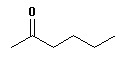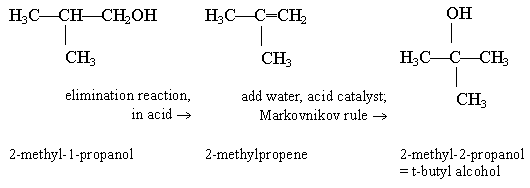## Quiz: Alcohols and ethers. Answer key.

1.2. a. ethyl propyl ether

b. ethoxypropane

In this case, a simple ether, the first name (part a) is probably more common. But it is good to be aware of naming alkoxy groups. The smaller group is (usually) the one referred to as an alkoxy group, attached to the larger main chain (Ouellette p 249).

c. 109 deg, the tetrahedral angle. There are two lone pairs on the O, thus four sets of electrons around it. This is always true for singly bonded O, in all molecules.

3. It's a phenol. At this point, you know about three types of O-containing functional groups (alcohols, phenols, ethers). Of these, only the phenol is acidic. Adding alcohols or ethers to water results in a neutral solution. (You will later meet one additional O-containing functional group that is acidic, the carboxyl group: -COOH. Ouellette Ch 12. But note that it has two O atoms.)

 4.This is 2-hexanone. (You are not formally responsible for naming ketones until Ch 10 in Ouellette.)

5. C4H10O + 6 O2 --> 4 CO2 + 5 H2O

6. a. One possible answer is dipropyl ether. (Any other ether with correct atoms is fine. For fun... how many possible answers are there here? Give it a try and e-mail me or slip me a note; include an explanation for your answer. I suggest you do part b first.)
b. No. The compound has 6 C and 14 H. Oxygen atoms do not affect the H count. Thus these compounds have the maximum possible number of H, and there can be no rings (or double bonds).
c. The alcohol. Alcohol molecules can H-bond with each other, because they have both H-bond donors and H-bond acceptors. Ethers are not H-bond donors; they do not have a polar H.

7.The simplest possible tertiary alcohol is 2-methyl-2-propanol. It is probably a good strategy to start this question by making that your target. It is then clear that the primary alcohol, the starting material, is 2-methyl-1-propanol. How do you "move" an -OH group? Take it off, and put it back. The second step follows Markovnikov.

8. This is a open-ended multi-part question. Most of the issues here have been raised in questions above, but this question allows you to tie many issues together in one context. Many answers are possible. I encourage you to show me what you did on this one, so I can give you feedback on it.

9. a. The basic reaction is the same as the one given in the question. We have just substituted one primary alcohol for another. The balanced equation shown will be the same for 1-butanol, in terms of moles Cr3+ per mole alcohol. The only difference is that the weight of a mole of alcohol is higher. If we made the calibration curve in terms of moles alcohol, it would be the same. With the normal calibration curve in terms of weight, we need to take into account the greater weight of the 1-butanol compared to the ethanol in the original equation.

b. Now we are using a secondary alcohol. There is an oxidation reaction, but it is different. The reaction with a primary alcohol involves two oxidation steps (primary alcohol --> aldehyde --> acid); the reaction here involves only one step (secondary alcohol --> ketone). Thus less Cr3+ (green color) will be made per mole alcohol. We will need to write a new equation, to determine the new ratio of mole Cr3+ to mole alcohol.

c. This is a tertiary alcohol; tertiary alcohols do not react with chromic acid, thus no analysis is possible.

This is probably a fairly difficult question for many X402 students. However, I should note that it builds on things that should be familiar to you. It uses the reactivity of different types of alcohols, from the current chapter. And it builds on your basic understanding of reaction stoichiometry, from general chemistry. The main difficulty probably arises from how many things you need to bring together at one time -- which is common in the real world. Balancing the oxidation equations would also be difficult, but I did not ask you to do that.

Last update: September 10, 2019